# ISO 216

﻿
ISO 216
ISO 269 sizes
(mm × mm)
C Series
C0 917 × 1297
C1 648 × 917
C2 458 × 648
C3 324 × 458
C4 229 × 324
C5 162 × 229
C6 114 × 162
C7/6 81 × 162
C7 81 × 114
C8 57 × 81
C9 40 × 57
C10 28 × 40
DLE 110 × 220
ISO 216 sizes
(mm × mm)
B Series
B0 1000 × 1414
B1 707 × 1000
B2 500 × 707
B3 353 × 500
B4 250 × 353
B5 176 × 250
B6 125 × 176
B7 88 × 125
B8 62 × 88
B9 44 × 62
B10 31 × 44
ISO 216 sizes
(mm × mm)
A Series
A0 841 × 1189
A1 594 × 841
A2 420 × 594
A3 297 × 420
A4 210 × 297
A5 148 × 210
A6 105 × 148
A7 74 × 105
A8 52 × 74
A9 37 × 52
A10 26 × 37

ISO 216 specifies international standard (ISO) paper sizes used in most countries in the world today. It defines the "A" and "B" series of paper sizes, including A4, the most commonly available size. Two supplementary standards, ISO 217 and ISO 269, define related paper sizes; the ISO 269 "C" series is commonly listed alongside the A and B sizes.

All ISO 216, ISO 217 and ISO 269 paper sizes have the same aspect ratio,$1:\sqrt{2}$. This ratio has the unique property that when cut or folded in half lengthwise, the halves also have the same aspect ratio. Each ISO paper size is one half of the area of the next size up.

## History

The advantages of basing a paper size upon an aspect ratio of √2 were already noted in 1786 by the German scientist Georg Christoph Lichtenberg, in a letter to Johann Beckmann. The formats that became A2, A3, B3, B4 and B5 were developed in France, and published in 1798 during the French Revolution, but were subsequently forgotten.[citation needed]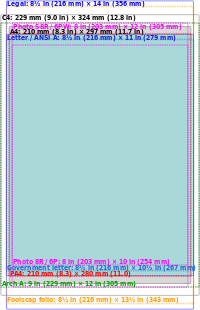Comparison of A4 (shaded grey) and C4 sizes with some similar paper and photographic paper sizes.

Early in the twentieth century, Dr Walter Porstmann turned Lichtenberg's idea into a proper system of different paper sizes. Porstmann's system was introduced as a DIN standard (DIN 476) in Germany in 1922, replacing a vast variety of other paper formats. Even today the paper sizes are called "DIN Ax" in everyday use in Germany and Austria.

The main advantage of this system is its scaling: if a sheet with an aspect ratio of √2 is divided into two equal halves parallel to its shortest sides, then the halves will again have an aspect ratio of √2. Folded brochures of any size can be made by using sheets of the next larger size, e.g. A4 sheets are folded to make A5 brochures. The system allows scaling without compromising the aspect ratio from one size to another – as provided by office photocopiers, e.g. enlarging A4 to A3 or reducing A3 to A4. Similarly, two sheets of A4 can be scaled down and fit exactly 1 sheet without any cutoff or margins.

The weight of each sheet is also easy to calculate given the basis weight in grams per square metre (g/m² or "gsm"). Since an A0 sheet has an area of 1 m², its weight in grams is the same as its basis weight in g/m². A standard A4 sheet made from 80 g/m² paper weighs 5g, as it is one 16th (four halvings) of an A0 page. Thus the weight, and the associated postage rate, can be easily calculated by counting the number of sheets used.

ISO 216 and its related standards were first published between 1975 and 1995:

• ISO 216:1975, defining the A and B series of paper sizes
• ISO 269:1985, defining the C series for envelopes
• ISO 217:1995, defining the RA and RSA series of raw ("untrimmed") paper sizes

## The A series

Paper in the A series format has a$1:\sqrt{2} \approx 0.707$ aspect ratio, although this is rounded to the nearest millimetre. A0 is defined so that it has an area of 1 square metre, prior to the above mentioned rounding. Successive paper sizes in the series (A1, A2, A3, etc.) are defined by halving the preceding paper size, cutting parallel to its shorter side (so that the long side of A(n+1) is the same length as the short side of An, again prior to rounding).

The most frequently used of this series is the size A4 which is 210 × 297 mm (8.3 × 11.7 in). For comparison, the letter paper size commonly used in North America (8.5" x 11") is approximately 6 mm (0.24 in) wider and 18 mm (0.71 in) shorter than A4.

The geometric rationale behind the square root of 2 is to maintain the aspect ratio of each subsequent rectangle after cutting the sheet in half, perpendicular to the larger side. Given a rectangle with a longer side, x, and a shorter side, y, the following equation shows how the aspect ratio of a rectangle compares to that of a half rectangle:$\ x/y = y/(x/2)$ which reduces to$x/y = \sqrt{2}$ or an aspect ratio of$1 : \sqrt{2}$.

The formula that gives the larger border of the paper size An in metres and without rounding off is the geometric sequence: an = 21 / 4 − n / 2. The paper size An thus has the dimension an × an + 1.

The exact millimetre measurement of the long side of An is given by$\left \lfloor 1000/(2^{\frac{2n-1}{4}})+0.2 \right \rfloor$.

## The B series

The B series are defined in the standard as follows: "A subsidiary series of sizes is obtained by placing the geometrical means between adjacent sizes of the A series in sequence." The use of the geometric mean means that each step in size: B0, A0, B1, A1, B2 … is smaller than the previous by an equal scaling. In a similar manner to the A series; the lengths of the B series still have the ratio$1:\sqrt{2}$, and folding one in half gives the next in the series. The shorter side of B0 is exactly 1m.

There is also an incompatible Japanese B series which the JIS defines to have 1.5 times the area of the corresponding JIS A series (which is identical to the ISO A series). Thus, the lengths of JIS B series paper are$\sqrt{1.5} \approx 1.22$ times those of A-series paper. By comparison, the lengths of ISO B series paper are$\sqrt{2} \approx 1.19$ times those of A-series paper.

For the ISO B series, the exact millimetre measurement of the long side of Bn is given by$\left \lfloor 1000/(2^{\frac{n-1}{2}})+0.2 \right \rfloor$.

## The C series

The C series formats are geometric means between the B series and A series formats with the same number, (e.g., C2 is the geometric mean between B2 and A2). The C series formats are used mainly for envelopes. An A4 page will fit into a C4 envelope. C series envelopes follow the same ratio principle as the A series pages. For example, if an A4 page is folded in half so that it is A5 in size, it will fit into a C5 envelope (which will be the same size as a C4 envelope folded in half).

A, B, and C paper fit together as part of a geometric progression, with ratio of successive side lengths of 21/8, though there is no size half-way between Bn and An-1: A4, C4, B4, "D4", A3, …; there is such a D-series in the Swedish extensions to the system.

The exact millimetre measurement of the long side of Cn is given by$\left \lfloor 1000/(2^{\frac{4n-3}{8}})+0.2 \right \rfloor$.

## Tolerances

The tolerances specified in the standard are:

• ±1.5 mm for dimensions up to 150 mm,
• ±2.0 mm for dimensions in the range 150 to 600 mm, and
• ±3.0 mm for dimensions above 600 mm.

## A, B, C comparison

ISO/DIN paper sizes in millimetres and in inches
A Series Formats B Series Formats C Series Formats
size mm inches mm inches mm inches
0 841 × 1189 33.1 × 46.8 1000 × 1414 39.4 × 55.7 917 × 1297 36.1 × 51.1
1 594 × 841 23.4 × 33.1 707 × 1000 27.8 × 39.4 648 × 917 25.5 × 36.1
2 420 × 594 16.5 × 23.4 500 × 707 19.7 × 27.8 458 × 648 18.0 × 25.5
3 297 × 420 11.7 × 16.5 353 × 500 13.9 × 19.7 324 × 458 12.8 × 18.0
4 210 × 297 8.3 × 11.7 250 × 353 9.8 × 13.9 229 × 324 9.0 × 12.8
5 148 × 210 5.8 × 8.3 176 × 250 6.9 × 9.8 162 × 229 6.4 × 9.0
6 105 × 148 4.1 × 5.8 125 × 176 4.9 × 6.9 114 × 162 4.5 × 6.4
7 74 × 105 2.9 × 4.1 88 × 125 3.5 × 4.9 81 × 114 3.2 × 4.5
8 52 × 74 2.0 × 2.9 62 × 88 2.4 × 3.5 57 × 81 2.2 × 3.2
9 37 × 52 1.5 × 2.0 44 × 62 1.7 × 2.4 40 × 57 1.6 × 2.2
10 26 × 37 1.0 × 1.5 31 × 44 1.2 × 1.7 28 × 40 1.1 × 1.6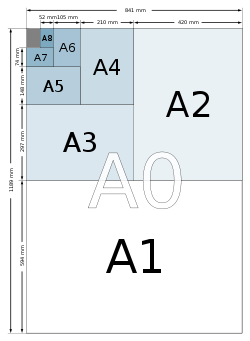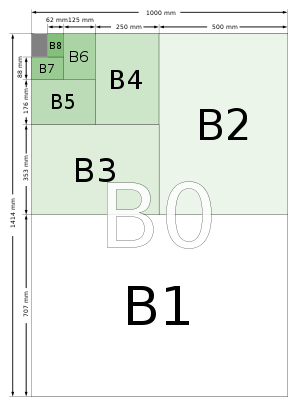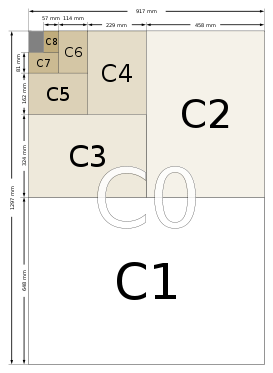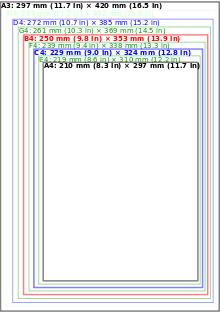Comparison of ISO 216 paper sizes between A4 and A3 and Swedish extension SIS 014711 sizes.

## Application

Before the adoption of ISO 216, many different paper formats were used internationally. These formats did not fit into a coherent system and were defined in terms of non-metric units.[citation needed]

The ISO 216 formats are organized around the ratio$1:\sqrt{2}$; two sheets next to each other together have the same ratio, sideways. In scaled photocopying, for example, two A4 sheets reduced to A5 size fit exactly onto one A4 sheet, and an A4 sheet in magnified size onto an A3 sheet, in each case there is neither waste nor want.

The principal countries not generally using the ISO paper sizes are the United States and Canada, which use the Letter, Legal and Executive system. Although they have also officially adopted the ISO 216 paper format, Mexico, The Philippines and Chile also use most U.S. paper sizes in ordinary usage.

Rectangular sheets of paper with the ratio$1:\sqrt{2}$ are popular in paper folding, where they are sometimes called "A4 rectangles" or "silver rectangles". However, in other contexts, the term "silver rectangle" can also refer to a rectangle in the proportion$1:(1+\sqrt{2})$, known as the silver ratio.

Wikimedia Foundation. 2010.

### Look at other dictionaries:

• Iso 216 — Dimensions ISO 216 (mm × mm) Séries A A0 841 × 1189 A1 594 × 841 A2 420 × 594 A3 297 × 420 A4 210 × 297 A5 148 × 210 A6 …   Wikipédia en Français

• ISO 216 — espceíficamente international standard (ISO) tamaño del papel, useda hoy en día en muchos países del mundo. Es el estándar que define el popular tamaño de papel A4 Las normas ISO 216 equivale a la DIN 476, y a las UNE 1011. Sustituciones en… …   Enciclopedia Universal

• ISO 216 — DIN EN ISO 216 Bereich Bürowesen Regelt Schreibpapier und bestimmte Gruppen von Drucksachen Endformate A und B Reihen und Kennzeichnung der Maschinenlaufrichtung …   Deutsch Wikipedia

• ISO 216 — Dimensions ISO 216 (mm × mm) Séries A A0 841 × 1189 A1 594 × 841 A2 420 × 594 A3 297 × 420 A4 210 × 297 A5 148 × 210 A6 …   Wikipédia en Français

• ISO 216 — Основная статья: Формат бумаги Международный стандарт ISO 216 определяет бумажные форматы. Он был создан в 1975 году из немецкого стандарта DIN 476 и отличается от него только большими допустимыми погрешностями. ISO 216:1975 определяет две серии… …   Википедия

• ISO 216 — Ilustración de la serie A. La norma ISO 216 de la Organización Internacional para la Estandarización (International Organization for Standardization, ISO) especifica los formatos de papel y es usada actualmente en muchos países del mundo. Es el… …   Wikipedia Español

• ISO 216:2007 — изд.2 E TC 6 Бумага писчая и некоторые виды печатной продукции. Форматы обрезанной полосы серий A и B и указание направления движения в машине раздел 85.080.10 …   Стандарты Международной организации по стандартизации (ИСО)

• EN ISO 216 — DIN EN ISO 216 Bereich Bürowesen Regelt Schreibpapier und bestimmte Gruppen von Drucksachen Endformate A und B Reihen und Kennzeichnung der Maschinenlaufrichtung …   Deutsch Wikipedia

• ISO 128 — is an international standard (ISO), about the general principles of presentation in technical drawings, more specific the graphical representation of objects on technical drawings. Contents 1 Overview 2 Composition of the ISO 128 …   Wikipedia

• ISO 217 — ISO 217:1995 стандарт определяющий серии бумажных форматов RA и SRA. Это серия форматов необрезанной «сырой» бумаги. RA означает «сырой формат А» (англ. “raw format A”), а SRA означает «дополнительный сырой формат A»… …   Википедия